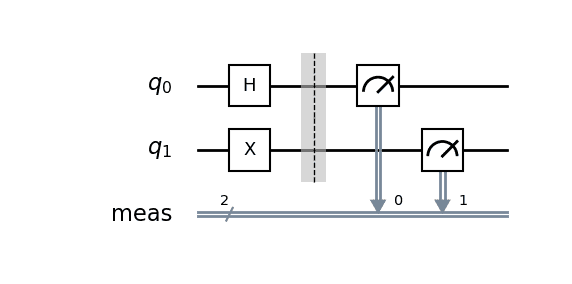# Extraction of a particular quantum state from a quantum circuit

ubergewehr273
TL;DR Summary
Created state ##\frac{1}{\sqrt{2}}(|01\rangle + e^{i\phi} |10 \rangle)## using a quantum circuit. I wish to further process this state to achieve ##\frac{1}{\sqrt{2}} (|01 \rangle + |10 \rangle)## in particular.
Hello everyone!

I'm trying to implement a quantum circuit that yields a superposition state $$\frac{1}{\sqrt{2}} (|01 \rangle + |10 \rangle)$$ I'm using parameterized gates to achieve this. I have been able to create the state $$\frac{1}{\sqrt{2}}(|01\rangle + e^{i\phi} |10 \rangle)$$ Is there a way to further process this state to achieve $$\frac{1}{\sqrt{2}} (|01 \rangle + |10 \rangle)$$ in particular?

Thanks!

Gold Member
2022 Award
This should be possible with a beam splitter and a phase shifter in one branch.

ubergewehr273
Thanks but I'm asking if some circuit components can be added somehow to process the former state to be the final state in question.

Heidi
Thanks but I'm asking if some circuit components can be added somehow to process the former state to be the final state in question.
How do you build your state? where is phi coming from?

ubergewehr273This is my reference circuit. This clearly produces the state ##\frac{1}{\sqrt{2}}(|01\rangle + |10\rangle)##. This is obviously until the measurement is done.

However, I'm trying to implement a circuit which essentially gives me a 50-50 chance of getting either ##|01\rangle## or ##|10\rangle##. And with this condition in mind, I am able to produce the state ##\frac{1}{\sqrt{2}}(|01\rangle + e^{i\phi}|10\rangle)## with some arbitrary ##\phi##. What I want to know if some further processing quantum gates can be done to produce the particular state with ##\phi = 0## $$\frac{1}{\sqrt{2}}(|01\rangle + |10\rangle)$$

Gold Member
Seems like a questions for @Strilanc ...

That said, I am still a bit confused about the question. The circuit you show already solves this problem; are you trying to come up with another implementation using parameterized gates?
Also, what is phi? It is obviously a phase, but where does it come from?

•vanhees71
Heidi
Seems like a questions for @Strilanc ...

That said, I am still a bit confused about the question. The circuit you show already solves this problem; are you trying to come up with another implementation using parameterized gates?
Also, what is phi? It is obviously a phase, but where does it come from?
Such a device would transform 01-10 into 01+10 (a bell state into another one)

Heidi
•vanhees71